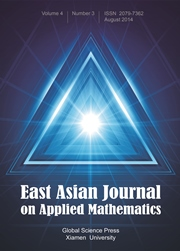Home
Hostname: page-component-55597f9d44-2qt69 Total loading time: 0.185 Render date: 2022-08-14T12:50:06.680Z Has data issue: true Feature Flags: { "shouldUseShareProductTool": true, "shouldUseHypothesis": true, "isUnsiloEnabled": true, "useRatesEcommerce": false, "useNewApi": true } hasContentIssue trueEast Asian Journal on Applied Mathematics

# Application of gPCRK Methods to Nonlinear Random Differential Equations with Piecewise Constant Argument

Published online by Cambridge University Press:  02 May 2017

*
*Corresponding author. Email addresses:cjzhang@mail.hust.edu.cn (C. Zhang), wenjieshihust@gmail.com (W. Shi)
*Corresponding author. Email addresses:cjzhang@mail.hust.edu.cn (C. Zhang), wenjieshihust@gmail.com (W. Shi)

## Abstract

We propose a class of numerical methods for solving nonlinear random differential equations with piecewise constant argument, called gPCRK methods as they combine generalised polynomial chaos with Runge-Kutta methods. An error analysis is presented involving the error arising from a finite-dimensional noise assumption, the projection error, the aliasing error and the discretisation error. A numerical example is given to illustrate the effectiveness of this approach.

## MSC classification

Type
Research Article
Information
East Asian Journal on Applied Mathematics , May 2017 , pp. 306 - 324

## Access options

Get access to the full version of this content by using one of the access options below. (Log in options will check for institutional or personal access. Content may require purchase if you do not have access.)

## References

 Babuska, I., Nobile, F. and Tempone, R., A stochastic collocation method for elliptic partial differential equations with random input data, SIAM J. Numer. Anal. 45, 10051034 (2007).CrossRefGoogle Scholar
 Beck, J., Nobile, F., Tamellini, L. and Tempone, R., Implementation of optimal Galerkin and collocation approximations of PDEs with random coefficients, ESIAM Proc. 33, 1021 (2011).CrossRefGoogle Scholar
 Ernst, O.G., Mugler, A., Starkloff, H.J. and Ullmann, E., On the convergence of generalized polynomial chaos expansions, ESIAM Math. Model. Numer. 46, 317339 (2012).CrossRefGoogle Scholar
 Ghanem, R. and Spanos, P., Stochastic Finite Elements: A Spectral Approach, Springer-Verlag, New York (1991).CrossRefGoogle Scholar
 Hairer, E., Nørsett, S. P. and Wanner, G., Solving Ordinary Differential Equations I: Nonstiff Problems, Springer, Berlin (1993).Google Scholar
 Liu, M., Ma, S. and Yang, Z., Stability analysis of Runge-Kutta methods for unbounded retarded differential equations with piecewise continuous arguments, Appl. Math. Comput. 191, 5766 (2007).Google Scholar
 Liu, X. and Liu, M., Asymptotic stability of Runge-Kutta methods for nonlinear differential equations with piecewise continuous arguments, J. Comput. Appl. Math. 280, 265274 (2015).CrossRefGoogle Scholar
 Narayan, A. and Zhou, T., Stochastic collocation on unstructured multivariate meshes, Commun. Comput. Phys. 18, 136 (2015).CrossRefGoogle Scholar
 Shi, W. and Zhang, C., Error analysis of generalized polynomial chaos for nonlinear random ordinary differential equations, Appl. Numer. Math. 62, 19541964 (2012).CrossRefGoogle Scholar
 Shi, W. and Zhang, C., Generalized polynomial chaos for nonlinear random Pantograph equations, Acta Math. Appl. Sin. English Ser. 32, 685700 (2016).CrossRefGoogle Scholar
 Tang, T. and Zhou, T., Recent developments in high order numerical methods for uncertainty quantification, Sci. Sin. Math. 45, 891928 (2015).Google Scholar
 Wang, W. and Li, S., Dissipativity of Runge-Kutta methods for neutral delay differential equations with piecewise constant delay, Appl. Math. Lett. 21, 983991 (2008).CrossRefGoogle Scholar
 Wang, W., Stability of solutions of nonlinear neutral differential equations with piecewise constant delay and their discretisations, Appl. Math. Comput. 219, 45904600 (2013).Google Scholar
 Wiener, J., Generalized Solutions of Functional Differential Equations, World Scientific, Singapore (1993).CrossRefGoogle Scholar
 Xiu, D., Fast numerical methods for stochastic computations: A review, Commun. Comput. Phys. 5, 242272 (2009).Google Scholar
 Xiu, D., Numerical Methods for Stochastic Computations: A Spectral Method Approach, Princeton University Press, Princeton, New Jersey (2010).Google Scholar
 Xiu, D. and Karniadakis, G.E., The Wiener-Askey polynomial chaos for stochastic differential equations, SIAM J. Sci. Comput. 24, 619644 (2002).CrossRefGoogle Scholar
 Zhou, T., A stochastic collocation method for delay differential equations with random input, Adv. Appl. Math. Mech. 6, 403418 (2014).CrossRefGoogle Scholar

# Save article to Kindle

Note you can select to save to either the @free.kindle.com or @kindle.com variations. ‘@free.kindle.com’ emails are free but can only be saved to your device when it is connected to wi-fi. ‘@kindle.com’ emails can be delivered even when you are not connected to wi-fi, but note that service fees apply.

Find out more about the Kindle Personal Document Service.

Application of gPCRK Methods to Nonlinear Random Differential Equations with Piecewise Constant Argument
Available formats
×

# Save article to Dropbox

To save this article to your Dropbox account, please select one or more formats and confirm that you agree to abide by our usage policies. If this is the first time you used this feature, you will be asked to authorise Cambridge Core to connect with your Dropbox account. Find out more about saving content to Dropbox.

Application of gPCRK Methods to Nonlinear Random Differential Equations with Piecewise Constant Argument
Available formats
×

# Save article to Google Drive

To save this article to your Google Drive account, please select one or more formats and confirm that you agree to abide by our usage policies. If this is the first time you used this feature, you will be asked to authorise Cambridge Core to connect with your Google Drive account. Find out more about saving content to Google Drive.

Application of gPCRK Methods to Nonlinear Random Differential Equations with Piecewise Constant Argument
Available formats
×
×